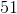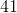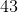# Common Core: 1st Grade Math : Understanding Place Value

## Example Questions

### Example Question #91 : Understanding Place Value

What digit is in the tens place?Explanation:

The tens place is always the second number from the right.

### Example Question #92 : Understanding Place Value

What digit is in the tens place?Explanation:

The tens place is always the second number from the right.

### Example Question #93 : Understanding Place Value

What digit is in the tens place?Explanation:

The tens place is always the second number from the right.

### Example Question #94 : Understanding Place Value

What digit is in the tens place?Explanation:

The tens place is always the second number from the right.

### Example Question #95 : Understanding Place Value

What digit is in the tens place?Explanation:

The tens place is always the second number from the right.

### Example Question #96 : Understanding Place Value

What digit is in the tens place?Explanation:

The tens place is always the second number from the right.

### Example Question #97 : Understanding Place Value

What digit is in the tens place?Explanation:

The tens place is always the second number from the right.

### Example Question #98 : Understanding Place Value

What digit is in the tens place?Explanation:

The tens place is always the second number from the right.

### Example Question #99 : Understanding Place Value

What digit is in the tens place?Explanation:

The tens place is always the second number from the right.

### Example Question #100 : Understanding Place Value

What digit is in the tens place?